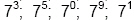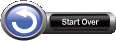Multiple Choice
Identify the choice that best completes the statement or answers the question.

1.

If Nicholas has \$3.87 already and earns \$7.92, how much does he have
altogether?
 a. \$1,179.00 d. \$10.79 b. \$4.05 e. \$11.95 c. \$11.79

2.

Measurement – Time, Temperature – RIT 201 – 210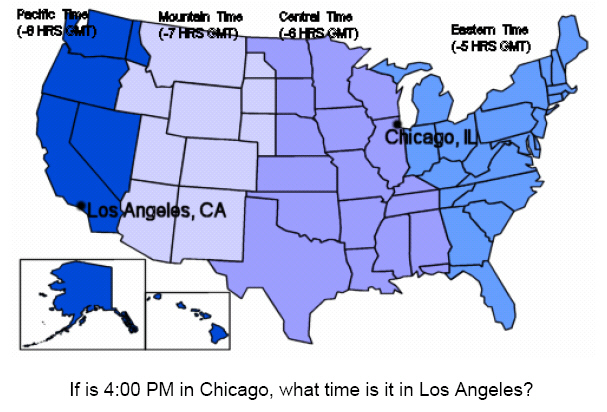a. 4:00 PM d. 3:00 PM b. 6:00 PM e. 5:00 PM c. 2:00 PM

3.

Number Sense and Numeration – Decimals – RIT 201 – 210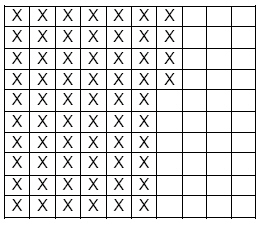How much of the square is shaded?
 a. 0.64 d. 64 b. 0.6 e. 0.36 c. 6.4

4.

What is 0.75 as a fraction?
 a. 1/4 d. 7/10 b. 1/2 e. 3/4 c. 2/3

5.

Round to the nearest tenth: 3.276
 a. 3.3 d. 3.28 b. 3.2 e. 3.27 c. 0.3

6.

2.378 x 100 =
 a. 0.2378 d. 237.8 b. 2.378 e. 23.78 c. 2378

7.

Number Sense and Numeration – Exponents – RIT 201 – 210a. 12 d. 256 b. 16 e. 4 c. 64

8.

Number Sense and Numeration – Fractions – RIT 201 –210
What is the simplest form of 15/20?
 a. 15/20 d. 1 5/20 b. 3/4 e. 5/20 c. 2/3

9.

____/18 = 2/3
 a. 9 d. 6 b. 10 e. 12 c. 15

10.

Name the fraction at the dot on the number line.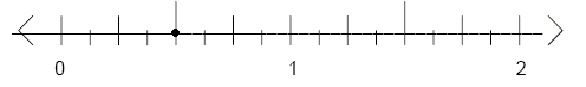a. 2/2 d. 3/4 b. 4/8 e. 5/4 c. 5

11.

Number Sense and Numeration – Ordering, Equalities and Inequalities –
RIT 201 – 210

Put the following numbers in order from least to greatest:
401, 210, 315, 211, 314, 423
 a. 210; 211; 314; 315; 401; 423 d. 210; 401; 211; 423; 314; 315 b. 423; 401; 315; 314; 211; 210 e. 211; 210; 315; 314; 423; 401 c. 401; 210; 211; 314; 315; 423

12.

Select the correct symbol for the box:

2,347,228,001 ____  2,347,598,001
 a. < d. > b. > e. = c. <

13.

Put the following numbers in order from least to greatest:
1/2, 0, -2/3, -3, 5
 a. 0; 1/2; -2/3; -3; 5 d. 5; -3; 1/2; -2/3; 0 b. –3; -2/3; 0; 1/2; 5 e. 0; -2/3; 1/2; -3; 5 c. 5; -3; -2/3; 1/2; 0

14.

Translate the following sentence into an equation:
“Twice a number, plus five, equals twenty-five.”
 a. 2(x + 5) = 25 d. x + 5 = 25 b. 2x – 5 = 25 e. x2 + 5 = 25 c. 2x + 5 = 25

15.

Put the following exponential values in order from least to greatest: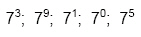a.d.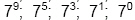b.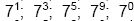e.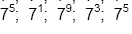c.